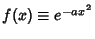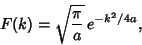## Fourier Transform--Gaussian

The Fourier Transform of a Gaussian Functionis given byThe second integrand is Even, so integration over a symmetrical range gives 0. The value of the first integral is given by Abramowitz and Stegun (1972, p. 302, equation 7.4.6)so a Gaussian transforms to a Gaussian.